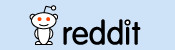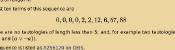mscroggs.co.uk
mscroggs.co.uksubscribe

Blog

Archive

Show me a random blog post
▼ show ▼
2013-07-11
On 19 June, the USB temperature sensor I ordered from Amazon arrived. This sensor is now hooked up to my Raspberry Pi, which is taking the temperature every 10 minutes, drawing graphs, then uploading them here. Here is a brief outline of how I set this up:

I found this code and adapted it to write the date, time and temperature to a text file. I then set cron to run this every 10 minutes. It writes the data to a text file (/var/www/temperature2) in this format:
2013 06 20 03 50,16.445019
2013 06 20 04 00,16.187843
2013 06 20 04 10,16.187843
2013 06 20 04 20,16.187843

Plotting the graphs

I found a guide somewhere on the internet about how to draw graphs with Python using Pylab/Matplotlib. If you have any idea where this could be, comment below and I'll put a link here.
In the end my code looked like this:
python
import time
import matplotlib as mpl
mpl.use("Agg")
import matplotlib.pylab as plt
import matplotlib.dates as mdates

ts = time.time()
import datetime
now = datetime.datetime.fromtimestamp(ts)
st = mdates.date2num(datetime.datetime(int(float(now.strftime("%Y"))),
int(float(now.strftime("%m"))),
int(float(now.strftime("%d"))),
0, 0, 0))
weekst = st - int(float(datetime.datetime.fromtimestamp(ts).strftime("%w")))
= file("/var/www/temperature2","r")
= []
= []
tt = []
ss = []
= []
= []
= []
= []
= []
weekt = []
weektt = []
weeks = []
weekss = []
mini = 1000
maxi = 0
cur = -1
datC = 0

for line in f:
fL = line.split(",")
fL = fL.split(" ")
if cur == -1:
cur = mdates.date2num(datetime.datetime(int(float(fL)),
int(float(fL)),
int(float(fL)),
0,0,0))
datC = mdates.date2num(datetime.datetime(int(float(fL)),
int(float(fL)),
int(float(fL)),
int(float(fL)),
int(float(fL)),
0))
u.append(datC)
v.append(fL)
if datC >= st and datC <= st + 1:
t.append(datC)
s.append(fL)
if datC >= st - 1 and datC <= st:
tt.append(datC + 1)
ss.append(fL)
if datC >= weekst and datC <= weekst + 7:
weekt.append(datC)
weeks.append(fL)
if datC >= weekst - 7 and datC <= weekst:
weektt.append(datC + 7)
weekss.append(fL)
if datC > cur + 1:
g.append(cur)
h.append(mini)
i.append(maxi)
mini = 1000
maxi = 0
cur = mdates.date2num(datetime.datetime(int(float(fL)),
int(float(fL)),
int(float(fL)),
0,0,0))
mini = min(float(fL),mini)
maxi = max(float(fL),maxi)
g.append(cur)
h.append(mini)
i.append(maxi)

plt.plot_date(x=t,y=s,fmt="r-")
plt.plot_date(x=tt,y=ss,fmt="g-")
plt.xlabel("Time")
plt.ylabel("Temperature (#^\circ#C)")
plt.title("Daily")
plt.legend(["today","yesterday"], loc="upper left",prop={"size":8})
plt.grid(True)
plt.gca().xaxis.set_major_formatter(mdates.DateFormatter("%H:%M"))
labels = plt.gca().get_xticklabels()
plt.setp(labels,rotation=90,fontsize=10)
plt.xlim(st,st + 1)
plt.savefig("/var/www/tempr/tg1p.png")

plt.clf()

plt.plot_date(x=weekt,y=weeks,fmt="r-")
plt.plot_date(x=weektt,y=weekss,fmt="g-")
plt.xlabel("Day")
plt.ylabel("Temperature (#^\circ#C)")
plt.title("Weekly")
plt.legend(["this week","last week"], loc="upper left",prop={"size":8})
plt.grid(True)
plt.gca().xaxis.set_major_formatter(mdates.DateFormatter("                     %A"))
labels = plt.gca().get_xticklabels()
plt.setp(labels,rotation=0,fontsize=10)
plt.xlim(weekst,weekst + 7)
plt.savefig("/var/www/tempr/tg2p.png")

plt.clf()

plt.plot_date(x=u,y=v,fmt="r-")
plt.xlabel("Date & Time")
plt.ylabel("Temperature (#^\circ#C)")
plt.title("Forever")
plt.grid(True)
plt.gca().xaxis.set_major_formatter(mdates.DateFormatter("%d/%m/%y %H:%M"))
labels = plt.gca().get_xticklabels()
plt.setp(labels,rotation=90,fontsize=8)
plt.savefig("/var/www/tempr/tg4p.png")

plt.clf()

plt.plot_date(x=g,y=h,fmt="b-")
plt.plot_date(x=g,y=i,fmt="r-")
plt.xlabel("Date")
plt.ylabel("Temperature (#^\circ#C)")
plt.title("Forever")
plt.legend(["minimum","maximum"], loc="upper left",prop={"size":8})
plt.grid(True)
plt.gca().xaxis.set_major_formatter(mdates.DateFormatter("%d %b"))
labels = plt.gca().get_xticklabels()
plt.setp(labels,rotation=90,fontsize=8)
plt.savefig("/var/www/tempr/tg3p.png")
If there's anything in there you don't understand, comment below and I'll try to fill in the gaps.

Finally, I upload the graphs to mscroggs.co.uk/weather. To do this, I set up pre-shared keys on the Raspberry Pi and this server and added the following as a cron job:
bash
0 * * * * scp /var/www/tempr/tg*p.png username@mscroggs.co.uk:/path/to/folder
I hope this was vaguely interesting/useful. I'll try to add more details and updates over time. If you are building something similar, please let me know in the comments; I'd love to see what everyone else is up to.
Edit: Updated to reflect graphs now appearing on mscroggs.co.uk not catsindrag.co.uk.

Similar postsHow OEISbot worksLogic botLogical contradictionsLogic bot, pt. 2